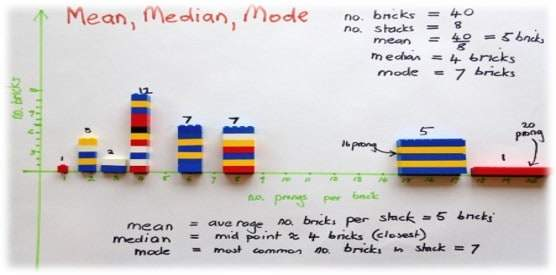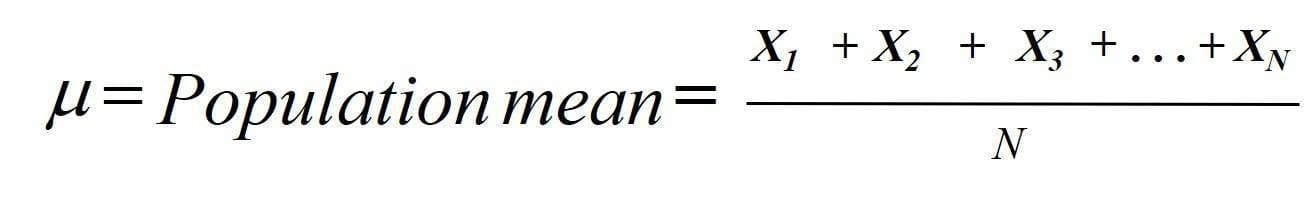Select Page## Using “Mean” as a Measures of Central Tendency

A measure of central tendency is a single value that describes the way in which a set of data cluster around a central value. In other words, it is a way to describe the center of a data set.

The three main measures of central tendency are:

### In this article we will discuss the Mean (or Average) of the Distribution

The mean is a measure of central tendency that considers all of the values in the data set. One of the limitations of the mean as a measure of central tendency is, in order to calculate the mean, the data must be numerical. You cannot use the mean when you are working with nominal data, which is data on attributes or characteristics. For example, there cannot calculate the mean of defective loan applications.

The mean is also called the arithmetic center of a distribution, stemming from the method to calculate the mean: the sum of all numbers in the data set, divided by how many numbers there are in the data set.For example, the mean of 1, 2, 3, 4, 5, 6 would be calculated:

[1+2+3+4+5+6] / 6 = 3.5

The mean takes all numbers of a set of data into account, which could be deemed as a strength of the measure, but this also means that it is susceptible to skewing of the final calculated figure if the data features extreme values (or outliers).

For example, in a data set – 1, 2, 3, 4, 25 – the mean would be 7

i.e. [1+2+3+4+25] / 2). This calculation of the mean of the distribution of data could be argued as unrepresentative as most values in the data set are smaller than 7. In this case the data set may take on a different shape.

### It’s important to know the shape of your data …

The mean is used to define the central location in a normally distributed set of data (where the shape is a normal or bell curve). Therefore, it is important for to understand the shape of data before describing the mean as a measure of central tendency.

I have sat in countless management meetings of companies that my team is engaged with and heard reports of average turn around times, average days to delivery, average throughput, etc.. I often ask the person reporting if using the “average” is the right measure for the report? Are they reporting the correct numbers? Do they know the shape of the data used for the report?When we look at the data through a statistical tool like Minitab, we may find that the data is not shaped like a bell curve but it s positively or negatively skewed. These shapes will lead us to report the median as the correct central tendency. The median in most cases will give a much different number than the mean.

I challenge you to question using the mean (or average) to describe the central tendency. Use a statistical tool like Minitab to learn the shape of the data. If the data shows normality, then the mean (or average) is the correct central tendency.LEARN THE 10 SIMPLE STEPS THAT WE TEACH LEAN SIX SIGMA GREEN BELTS TO GET AMAZING RESULTS FROM THEIR PROJECTS.